# What is I/Q Data?

Publish Date: Oct 16, 2019 | 337 Ratings | 4.32 out of 5 | Print | 34 Customer Reviews | Submit your review

## Overview

This white paper is part of the NI Measurement Fundamentals main page series. Each page in this series teaches you a specific concept related to common measurement applications by explaining the theory and giving practical examples. This white paper describes the theoretical background of I/Q data as well as practical considerations which make the use of I/Q data in communication so desirable.

Put simply, I/Q data shows the changes in magnitude (or amplitude) and phase of a sine wave. If amplitude and phase changes occur in an orderly, predetermined fashion, you can use these amplitude and phase changes to encode information upon a sine wave, a process known as modulation.

Modulation changes a higher frequency carrier signal in proportion to a lower frequency message, or information, signal. I/Q data is highly prevalent in RF communications systems, and more generally in signal modulation, because it is a convenient way to modulate signals.

### 1. Background on Signals

Signal modulation changes a sine wave to encode information. The equation representing a sine wave is as follows: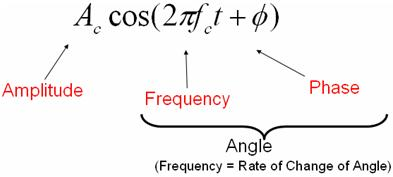Figure 1: Equation for a Sine Wave

The equation above shows that you are limited to making changes to the amplitude, frequency, and phase of a sine wave to encode information. Frequency is simply the rate of change of the phase of a sine wave (frequency is the first derivative of phase), so frequency and phase of the sine wave equation can be collectively referred to as the phase angle. Therefore, we can represent the instantaneous state of a sine wave with a vector in the complex plane using amplitude (magnitude) and phase coordinates in a polar coordinate system.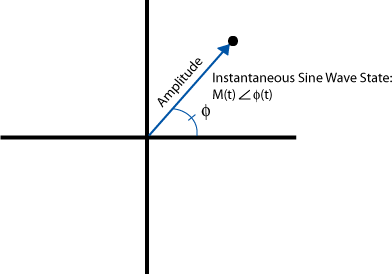Figure 2. Polar Representation of a Sine Wave

In the graphic above, the distance from the origin to the black point represents the amplitude (magnitude) of the sine wave, and the angle from the horizontal axis to the line represents the phase. Thus, the distance from the origin to the point remains the same as long as the amplitude of the sine wave is not changing (modulating). The phase of the point changes according to the current state of the sine wave. For example, a sine wave with a frequency of 1 Hz (2π radians/second) rotates counter-clockwise around the origin at a rate of one revolution per second. If the amplitude doesn't change during one revolution, the dot maps out a circle around the origin with radius equal to the amplitude along which the point travels at a rate of one cycle per second.

Because phase is a relative measurement, imagine that the phase reference used is a sine wave of frequency equal to the sine wave represented by the amplitude and phase points. If the reference sine wave frequency and the plotted sine wave frequency are the same, the rate of change of the two signals' phase is the same, and the rotation of the sine wave around the origin becomes stationary. In this case, a single amplitude/phase point can represent a sine wave of frequency equal to the reference frequency. Any phase rotation around the origin indicates a frequency difference between the reference sine wave and the sine wave being plotted.

Up to this point, this white paper has described amplitude and phase data in a polar coordinate system. All the concepts discussed above apply to I/Q data. In fact, I/Q data is merely a translation of amplitude and phase data from a polar coordinate system to a Cartesian (X,Y) coordinate system. Using trigonometry, you can convert the polar coordinate sine wave information into Cartesian I/Q sine wave data. These two representations are equivalent and contain the same information, just in different forms. This equivalence is show in Figure 3.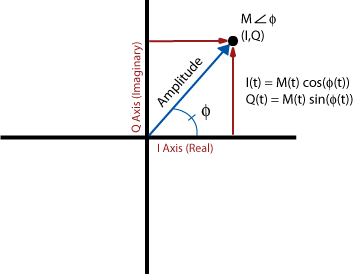Figure 3. I and Q Represented in Polar Form

The figure below shows a LabVIEW example demonstrating the relationship between polar and Cartesian coordinates.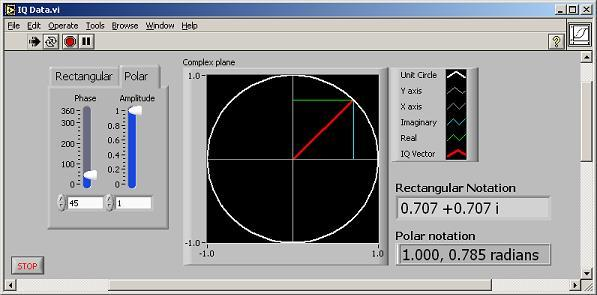Figure 4: I/Q Data in LabVIEW

### 2. I/Q Data in Communication Systems

To explain why I/Q data is used in communications systems, you must understand modulation basics.

RF communication systems use advanced forms of modulation to increase the amount of data that can be transmitted in a given amount of frequency spectrum. Signal modulation can be divided into two broad categories: analog modulation and digital modulation. Analog or digital refers to how the data is modulated. If analog audio data is modulated onto a carrier sine wave, this technology is referred to as analog modulation. If analog audio data is sampled by an analog-to-digital converter (ADC) with the resulting digital bits modulated onto a carrier sine wave, this technology is defined as digital modulation because digital data is encoded. Both analog modulation and digital modulation involve changing the carrier wave amplitude, frequency, or phase (or combination of amplitude and phase simultaneously) according to the message data.

Amplitude modulation (AM), frequency modulation (FM), or phase modulation (PM) are all examples of analog modulation.  With amplitude modulation, the carrier sine wave amplitude is modulated according to the message signal. The same idea holds true for frequency and phase modulation.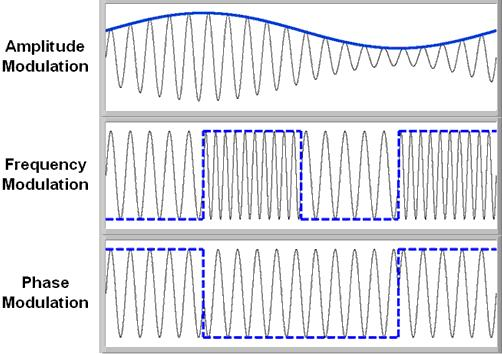Figure 5. Time Domain of AM, FM, and PM Signals

Figure 5 represents various analog techniques—AM, FM, and PM—applied to a carrier signal. For AM, the message signal is the blue sine wave that forms the "envelope" of the higher frequency carrier sine wave. For FM, the message data is the dashed square wave. As the figure illustrates, the resulting carrier signal changes between two distinct frequency states. Each  frequency state represents the high and low state of the message signal. If the message signal were a sine wave in this case, there would be a more gradual change in frequency, which would be more difficult to see. For PM, notice the distinct phase change at the edges of the dashed square wave message signal.

As mentioned earlier, if only the carrier sine wave amplitude changes with respect to time (proportional to the message signal), as is the case with AM modulation, the I/Q plane graph changes only with respect to the distance from the origin to the I/Q points, as shown in the following image: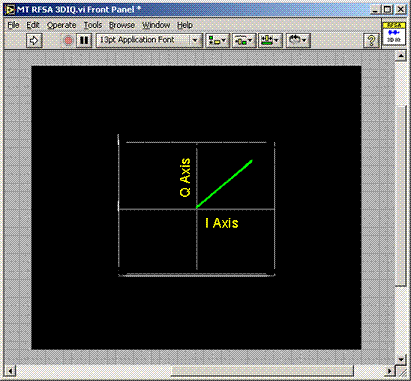Figure 6. I/Q Data in the Complex Domain

The preceding figure shows the I/Q data points vary in amplitude only, with the phase fixed at 45 degrees. You cannot tell much about the message signal, only that it is amplitude modulated. However, if you watch how the I/Q data points vary in magnitude with respect to time, you can essentially see a representation of the message signal. Using LabVIEW's 3D graph control, we can show the third axis of time to illustrate the message signal.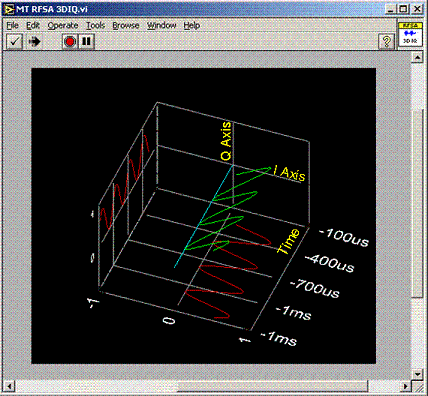Figure 7. Representation of Magnitude vs. Time

Figure 7 shows the same data as the 2D I vs. Q plot in Figure 6. The magnitude of the signal trace modulates in a sinusoidal pattern, indicating that the message signal is a sine wave. The green trace represents the amplitude and phase data in a polar coordinate system, while the red traces represent the projections of this waveform onto the I and Q axes, representing the individual I and Q waveforms.

We can show the same type of example using PM, as shown in the following figure: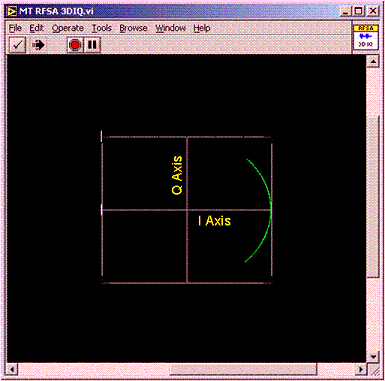Figure 8. Polar Representation of Phase vs. Time

You can tell that the message signal is phase modulated, as the amplitude is constant but the phase is changing (modulating). You cannot see the shape of the message signal with respect to time, but you can see the minimum and maximum signal levels of the message signal are represented by phase deviations of -45 degrees and +45 degrees respectively.

The time axis can be used to better understand this concept, as shown in the following figure: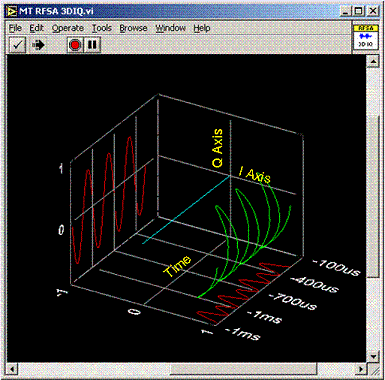Figure 9. 3D Representation of Phase Modulation

Figure 9, shown in the LabVIEW 3D graph, shows the green trace varying in a sinusoidal fashion with respect to time. The projections onto the I and Q axes represent the individual I and Q waveforms corresponding to the PM sine wave with fixed magnitude and oscillating phase.

In essence, the I/Q data represents the message signal. Because the I/Q data waveforms are Cartesian translations of the polar amplitude and phase waveforms, you may have trouble determining the nature of the message signal. For example, compare the red I and Q traces on the 3D I vs. Q plots in Figure 9 to the green trace in Figure 9. If you plot amplitude vs. time for the AM sine wave, you would see the message signal. If you plot the phase data vs. time for the AM sine wave, you would have a straight line. You would see sine waves for the I vs. time and Q vs. time waveforms as well, but the scale would be off, and this would not necessarily be the case for more complex digital modulation schemes where both amplitude and phase are modulated simultaneously.

### 3. So Why Use I/Q Data?

Because amplitude and phase data seem more intuitive, you might assume you should use polar amplitude and phase data instead of Cartesian I and Q data. However, practical hardware design concerns make I and Q data the better choice.

Precisely varying the phase of a high-frequency carrier sine wave in a hardware circuit according to an input message signal is difficult. A hardware signal modulator that manipulates the amplitude and phase of a carrier sine wave would therefore be expensive and difficult to design and build, and, as it turns out, not as flexible as a circuit that uses I and Q waveforms. To understand how to avoid manipulating the phase of an RF carrier directly, refer to the following I/Q modulation equations: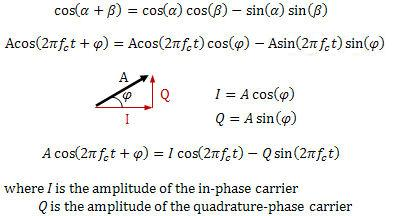Figure 10. Mathematical Background of I/Q Modulation

According to the trigonometric identity shown in the first line of Figure 10, multiply both sides of the equation by A and substitute 2πfct in place of α and φ in place of β to arrive at the equation shown in line 2. Then substitute I for A cos(φ) and Q for A sin(φ) to represent a sine wave with the equation shown on line 3.

Remember that the difference between a sine wave and a cosine wave of the same frequency is a 90-degree phase offset between them. Essentially, what this fact means is that you can control the amplitude, frequency, and phase of a modulating  carrier sine wave by simply manipulating the amplitudes of separate I and Q input signals. With this method, you do not need to directly vary the phase of an RF carrier sine wave. You can achieve the same effect by manipulating the amplitudes of input I and Q signals. Of course, the second half of the equation is a sine wave and the first half is a cosine wave, so you must include a device in the hardware circuit to induce a 90-degree phase shift between the carrier signals used for the I and Q mixers, but this addition is a simpler design issue than the aforementioned direct phase manipulation.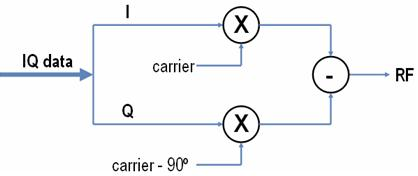Figure 11. Hardware Diagram of an I/Q Modulator

Figure 11 shows a block diagram of an I/Q modulator. The circles with an 'X' represent mixers—devices that perform frequency multiplication and either upconvert or downconvert signals (upconverting here). The I/Q modulator mixes the I waveform with the RF carrier sine wave, and it mixes the Q signal with the same RF carrier sine wave at a 90-degree phase offset. The Q signal is subtracted from the I signal (just as in the equation shown in line 3 in Figure 10) producing the final RF modulated waveform. In fact, the 90-degree shift of the carrier is the source of the names for the I and Q data—I refers to in-phase data (because the carrier is in phase) and Q refers to quadrature data (because the carrier is offset by 90 degrees). This technique is known as quadrature upconversion, and you can use the same I/Q modulator for any modulation scheme. The I/Q modulator is merely reacting to changes in I and Q waveform amplitudes, and I and Q data can represent any changes in magnitude and phase of a message signal. The flexibility and simplicity (relative to other options) of the design of an I/Q modulator is why it is so widely used and popular.

### 5. Conclusions

This document is meant to provide a brief overview and introduction to I/Q data as it relates to RF and wireless systems. For the complete list of tutorials, return to the NI Measurement Fundamentals main page, or for more RF tutorials, refer to the NI RF Fundamentals page.  Additional information can also be found with Teaching and Research Resources for RF and Communications and the NI-RFSA help file.

Customer Reviews
34 Reviews | Submit your review

representation  - Jul 28, 2016

the representattion of sine wave in figure 6 and 7 is bit confussing. while the sine wave is made up of complete cycle of 360 degree in the sence that complete rottion of a circle in polar form. how can we in figure 7 represent a sine wave just for 1 phase value of 45 degree.?

I loved it! Need some more examples with some examples and pros and cons  - Feb 4, 2016

Its an excellent starter for fundamental understanding. I would like to have some more examples as well as more insight into the issues associated with this and how the industry is dealing with them as well as sources of IQ imbalance.

I don't see how modulating I & Q can modulate the frequency  - Dec 22, 2015

You've shown how modulating the amplitude of the I and Q can modulate the phase and amplitude of the final carrier transmitted, but you haven't shown how frequency modulation is achieved. Yet you state "what this fact means is that you can control the amplitude, frequency, and phase of a modulating carrier sine wave by simply manipulating the amplitudes of separate I and Q input signals. "

Its very simple, clean and clear for the first time reader  - Feb 18, 2013

Its very simple, clean and clear for the first time reader

Magnitude is equivalent to Amplitude?  - Apr 29, 2012

Hi! A[c] is the magnitude of the vector that represents a point on the amplitude vs. time graph, correct? Thus, A[c] = sqrt (Amplitude**2 + time**2) Where Amplitude is voltage. Is that correct?

Great Tutorial!!  - Sep 17, 2011

Great Tutorial about I/Q data I have ever read!! Go Go NI!!

simple and efficient   - Jan 4, 2011

The tutorial was very useful especially the equations which proved how IQ data can be used for simultaneous amplitude and phase modulation. Good job and thanks a lot

- Dec 20, 2010

Great Tutorial! Thanks!

sudar  - Jul 10, 2010

How can u change amplitude freq and phase by just changing the amplitude only of the I and q signals? clarity is missing in important places. But I guess this doc's scope is not the details.

- Jun 25, 2010

Wow. Very good. I look forward to learning the next step now that I understand the IQ basics. I've seen the crazy IQ plots from downconverted signals and always wondered how to interpret them. I'm eager for the next topic.

- May 26, 2010

Always a good job!

Great tutorial  - Apr 25, 2010

Clear, cohincise and precise. In five minutes I finally grabbed the basics of I/Q. Do keep the great tutorial work!!

- Apr 8, 2010

its very useful,i was searching this details for 2 days,here u have every thing so clearly good job

Slight confusion  - Nov 1, 2009

In the discussion following Fig. 5 the modulations shown are described as "analog," whereas the FM and PM modulations have two states - are digital.

Another technical overview on generating I/Q data  - Apr 27, 2009

This document on I/Q data is excellent. However it will be nice if there is another article on how to generate I/Q data for FSK, PSK or GMSK of a message stream.

Equation error (typo)  - Dec 4, 2008

Superb article, but I believe equation 2, which was derived from equation 1 multiplied through by A, is missing the A from the right-most term. However, later in the final equation, it choses to include this A in the substitution for Q, so it ends up just being a simple typo on equation 2. Congratulations though on an absolutely first rate piece of work.

Great instruction, just one more addition  - Jul 28, 2008

It is not clear from the text how do we actually can generate I and Q data from the Acos(w_c*t+phi), i.e, a figure for transmitter side similar to Fig 9 for the receiver side is missing. I spent 20 more mins and figured out that if we simply multiply the signal by cos(w_c*t) and -sin(w_c*t), followed by a LPF, we can generate the I and Q data for transmission.

Simple and clever  - Jun 18, 2008

I'm a telecoms engineer who almost forgot where the hell the I/Q come from! With this simple and clever explanation its better to remember.

Excellent!  - Apr 16, 2008

Great explanation...better than most texts!

Wow, finally I understand  - Mar 22, 2008

Great explanation! As a mechanical engineer, I've been trying to understand this EE principle forever. Now I do.

Excellent easy to understand  - Feb 21, 2008

I train electronic technicians for the AF and trying to explain many concepts like !/Q, FFT, and digital mod types is often difficult at best. Your text and supporting images are thorough yet simple enough that even I can understand. Now I have some great basics to use in describing these principles to new technicians.

- Feb 9, 2008

I am a student of Texas A & M ,very simple to understand.

IQ is now clear for me!  - Jun 27, 2007

Many thanks for the clear explanation of IQ signals! Rudolf

Concise  - Jun 21, 2007

I enjoyed the clarity, simplicity and lack of vocab words. This is the first clear explanation of IQ I have seen. RF engineering need not be "black magic".

Perfect explaination with practical application  - Apr 27, 2007

This is very good explaination of pratical concern..why it is used.. no jorgan in understanding this

Perfect explaination with practical application  - Apr 27, 2007

This is very good explaination of pratical concern..why it is used.. no jorgan in understanding this

- Apr 8, 2007

Extremely good explanation. Thank you

Very clear, useful and helpful  - Apr 3, 2007

It just saved my day.... and night~!

Keep up the Good Work..  - Feb 24, 2007

very simple and intuitive...

- Jan 24, 2007

Very clear explanation.

Trig identity omitted  - Jan 18, 2007

Minor quibble: In Figure 9, you omitted the trig identity referred to as "line 1" in the text, i.e. cos(alpha+beta)=cos(alpha) cos(beta) - sin(alpha)sin(beta). Also, you didn't number the lines referred to in the text as lines 2 and 3. But very enlightening all the same!

EXCELLENT!!!!!!!  - Dec 7, 2006

It took me 30 minutes only to understand IQ !!! And before that I wasted one complete week .

Clear, Consise, perfect summary  - Dec 7, 2006

Pictures / graphs always help better your understanding. I am a novice on RF principles. This tutorial on I/Q mod/demod is as good as one can make for a beginner.

Finally any excellent and simple description of what I/Q data is!  - Nov 19, 2006

For the first time, i finally understood what I/Q means!